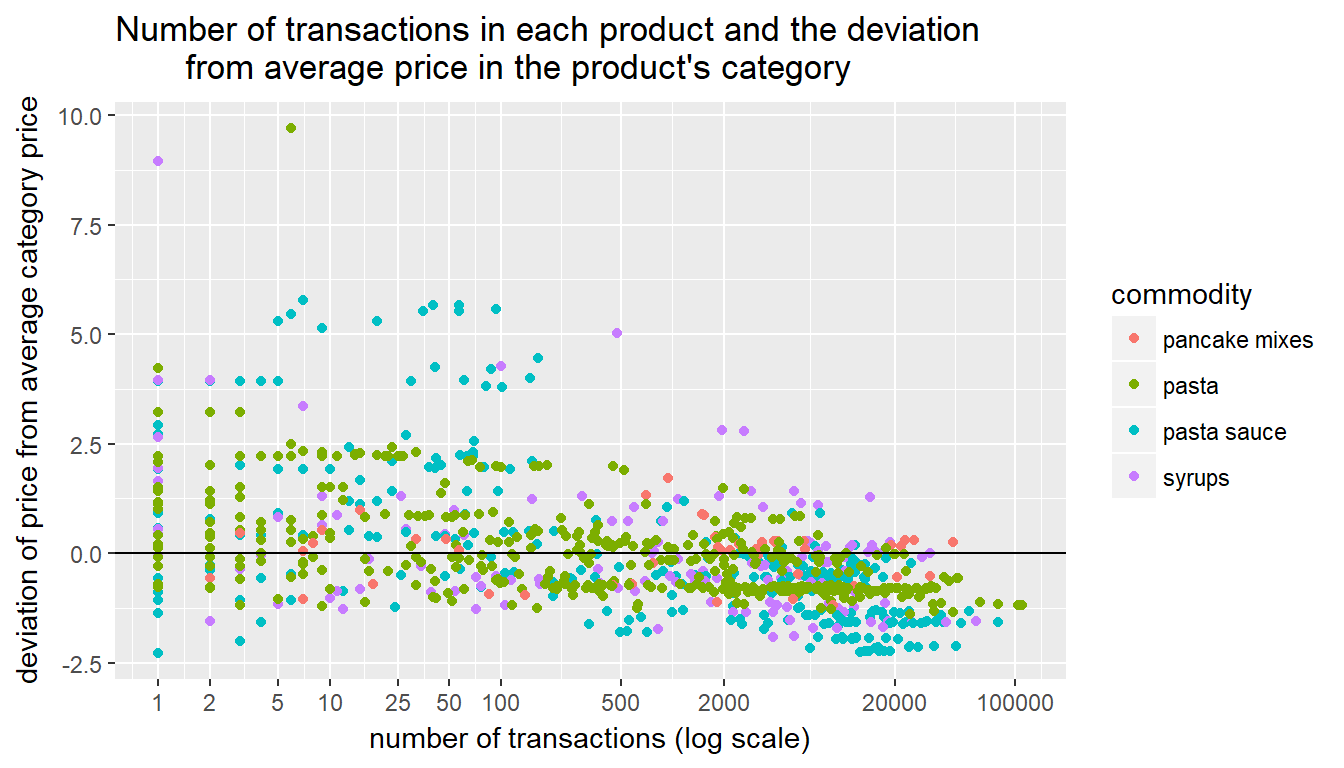# Lab 6: Summarizing data within groups using group_by() and mutate()

### Learning Objectives

• calculating within groups statistics using group_by() and mutate()
• learning when to apply mutate() and when to apply summarize()

### 0. Introduction

Let’s load in our prodsales data from Lab 5.

library(tidyverse)
prodsales <- read_csv("prodsales.csv")

### 1. Calculating within groups statistics using group_by() and mutate()

Our prodsales data has the average price for each product. Since some product categories, such as pasta sauce, tend to be more expensive than others, such as pasta, let’s calculate how prices of individual products differ from the average prices their product category. This means that we need to calculate average price within a category, but we don’t want the data to collapse. We want a new variable in prodsales that has the average price in each category. We can accomplish this using functions group_by() and mutate(). This is because mutate() calculates summary statistics within groups defined by group_by().

prodsales <- prodsales %>% group_by(commodity) %>% mutate(av_cat_price=mean(av_price))
head(select(prodsales, product_description, commodity, av_price, av_cat_price), n=5)
## # A tibble: 5 x 4
## # Groups: commodity 
##   product_description       commodity   av_price av_cat_price
##   <chr>                     <chr>          <dbl>        <dbl>
## 1 KARO RED SYRUP            syrups         12.0          3.04
## 2 KRAFT 5 PK MAC & CHEESE   pasta          11.5          1.77
## 3 PATTY PIZZAILO SAUCE      pasta sauce     8.85         3.06
## 4 PATSYS PUTTANESCA SCE 24Z pasta sauce     8.74         3.06
## 5 PATSYS TOMATO BASIL SAUCE pasta sauce     8.73         3.06

Notice that we grouped data by product category (variable commodity). The number of observations is still 926. We also see that av_cat_price is the same in rows that have the same category. Let’s calculate how each product’s price deviates from the average price in its category. Then, let’s plot this deviation against number of transactions. We may want to use log scale for the number of transactions. Also, to make the graph more informative let’s vary the color by product category.

prodsales$price_dev <- prodsales$av_price - prodsales$av_cat_price ggplot(prodsales, aes(y=price_dev,x=no_trans,color=commodity)) + geom_point() + scale_x_continuous(trans="log", breaks=c(1, 2, 5, 10, 25, 50, 100,500,2000, 20000,100000)) + labs(title="Number of transactions in each product and the deviation from average price in the product's category") + ylab("deviation of price from average category price") + xlab("number of transactions (log scale)") + geom_hline(aes(yintercept=0))It is pretty clear that relatively lower priced items have somewhat higher volume of transactions. We can also detect somewhat higher price elasticity of demand for pasta than for pasta sauce. ### IN-CLASS EXERCISE: 1. Calculate the market share of each brand in its product category. Create a data frame with top three brands in each category. 2. Suppose we wanted to predict the outcome of game in our NHL data set. What variables would you want to use? 3. (cumsum() function) Suppose you wanted to create a measure of the opposing teams success so far in the season. Suppose further that you wanted to measure that success by counting the number of games won so far in the season. Create a variable that calculates that number for each team (home and visiting) for each game. The code below is to get you started. Can you tell what it does? nhl <- read_csv("NHLseason2016.csv") home_games <- nhl %>% rename(team=Home, goals_for=goals_home, goals_against=goals_visit) %>% select(Date, team, goals_for, goals_against) away_games <- nhl %>% rename(team=Visitor, goals_for=goals_visit, goals_against=goals_home) %>% select(Date, team, goals_for, goals_against) games <- bind_rows(home_games, away_games) games$win <- ifelse(games$goals_for-games$goals_against>0,1,0)
games <- arrange(games, team, Date)

### Exercises:

In solving the type of problems below you normally need to make three decision: First, decide whether you need to collapse the data or just calculate a new column based on some groups (i.e. summarize vs. mutate). Second, decide by which group or groups you want to collapse or make a calculations (i.e. which variable(s) go into group_by()). Finally, decide what calculation(s) need to happen when you collapse or mutate (i.e. which functions, =sum(), =mean(), =n() etc. go inside summarize or mutate).

1. Load in dunnhumby’s trans data and filter out negative sales. Load in the prods data as well. Fix the upc code in prods so that it is a 10-digit character with leading zeros where needed. We did this in lab 5.

2. How many different brands exist in each product category? (Hint 1: You will need to collapse data twice. Hint 2: It is possible to collapse data without making any calculations. For example, df2 <- df %>% group_by(a,b) %>% summarize() will return columns a and b and one row for each combination of values in a and b that exists in df.)

3. Let’s examine brand loyalty. As a first step, let’s focus on transactions from frequent buyers in each category. Create data that has only transactions from households and product categories in which the household made at least 10 transactions in that product category. How many transactions did we lose by eliminating infrequent buyers? (Hint: Since transaction data only has upc but not product category, you will need to merge-in product category into the transaction data.)

4. Among households who bought at least 10 items in a product category (i.e. the data you created in the previous question), what is the median number of brands in each product category that households buy? (Hint 1: You will need to “collapse” your data three times. Hint 2: The medium number of brands of pasta that households buy is 4.)

5. Would you say that brand loyalty varies by product category?